# Electronics and Communication Engineering - Analog Electronics

16.

Values of VT at 20°C for an ideal P-N diode

 A. 25.27 mV B. 30.3 mV C. 23 mV D. 50 mV

Explanation: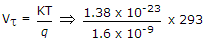.

17.

Assertion (A): In an op-amp the voltage gain and band width can be adjusted as per requirement

Reason (R): Large value capacitor can also be fabricated on a chip

 A. Both A and R are correct and R is correct explanation for A B. Both A and R are correct but R is not correct explanation for A C. A is correct R is wrong D. A is wrong R is correct

Explanation:

Large value capacitor cannot be fabricated on a chip.

18.

In figure which diode will conduct and what will be the value of V0?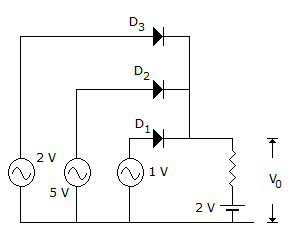A. D3, 1 V B. D1, 2 V C. D2, 5 V D. D1, 5 V

Explanation:

Since battery connected to anode of D, is of 5 V it is forward biased. D1 and D3 are reverse biased. Output voltage is 5 V.

19.

For the amplifier circuit of figure, the h parameters of transistor are hib = 25 Ω, hfb = 0.999, hob = 10-6 Ω. The voltage gain is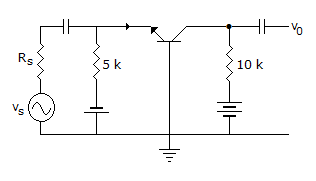A. 0.999 B. 1.98 C. 20 D. 400

Explanation:

It is a common base circuit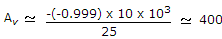.

20.

In figure, VCC = + 30 V, R1 = 200 kΩ and R2 = 100 kΩ. If VBE = 0.7 V, the voltage a cross RE =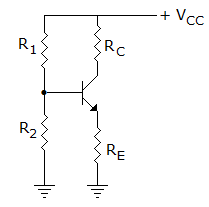A. +20 V B. +19.3 V C. +10 V D. +9.3 V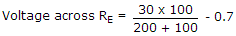.